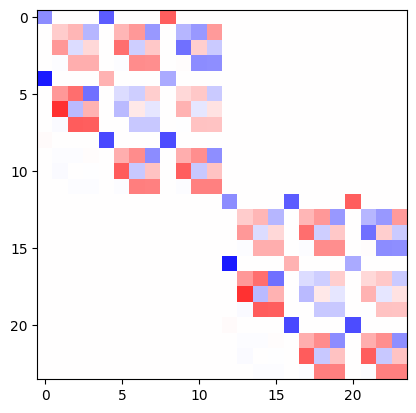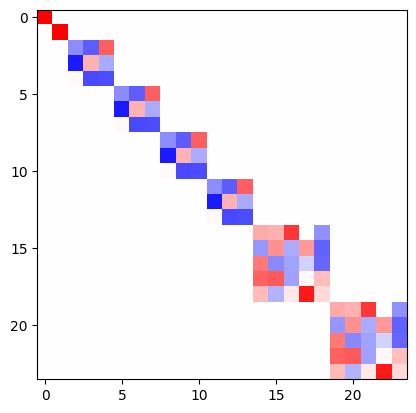# Euclidean neural networks

## What is e3nn?

e3nn is a python library based on pytorch to create equivariant neural networks for the group $$O(3)$$.

## Demonstration

All the functions to manipulate rotations (rotation matrices, Euler angles, quaternions, convertions, …) can be found here Parametrization of Rotations. The irreducible representations of $$O(3)$$ (more info at Irreps) are represented by the class e3nn.o3.Irrep. The direct sum of multiple irrep is described by an object e3nn.o3.Irreps.

If two tensors $$x$$ and $$y$$ transforms as $$D_x = 2 \times 1_o$$ (two vectors) and $$D_y = 0_e + 1_e$$ (a scalar and a pseudovector) respectively, where the indices $$e$$ and $$o$$ stand for even and odd – the representation of parity,

import torch
from e3nn import o3

irreps_x = o3.Irreps('2x1o')
irreps_y = o3.Irreps('0e + 1e')

x = irreps_x.randn(-1)
y = irreps_y.randn(-1)

irreps_x.dim, irreps_y.dim

(6, 4)


their outer product is a $$6 \times 4$$ matrix of two indices $$A_{ij} = x_i y_j$$.

A = torch.einsum('i,j', x, y)
A

tensor([[-1.5060,  0.5162, -0.6796, -1.6781],
[ 0.2336, -0.0801,  0.1054,  0.2603],
[-0.3430,  0.1176, -0.1548, -0.3822],
[ 0.3441, -0.1180,  0.1553,  0.3835],
[ 0.6740, -0.2310,  0.3042,  0.7510],
[ 1.3570, -0.4652,  0.6124,  1.5121]])


If a rotation is applied to the system, this matrix will transform with the representation $$D_x \otimes D_y$$ (the tensor product representation).

$A = x y^t \longrightarrow A' = D_x A D_y^t$

Which can be represented by

R = o3.rand_matrix()
D_x = irreps_x.D_from_matrix(R)
D_y = irreps_y.D_from_matrix(R)

plt.imshow(torch.kron(D_x, D_y), cmap='bwr', vmin=-1, vmax=1);This representation is not irreducible (is reducible). It can be decomposed into irreps by a change of basis. The outerproduct followed by the change of basis is done by the class e3nn.o3.FullTensorProduct.

tp = o3.FullTensorProduct(irreps_x, irreps_y)
print(tp)

tp(x, y)

FullTensorProduct(2x1o x 1x0e+1x1e -> 2x0o+4x1o+2x2o | 8 paths | 0 weights)

tensor([ 0.1382,  0.9805, -1.5060,  0.2336, -0.3430,  0.3441,  0.6740,  1.3570,
0.2935,  1.2698, -0.4240,  0.0980, -0.6001,  0.2732, -1.1035, -0.5372,
0.0314,  0.0746, -0.6353, -0.0578, -0.0535, -0.3208,  0.9641,  1.1527])


As a sanity check, we can verify that the representation of the tensor prodcut is block diagonal and of the same dimension.

D = tp.irreps_out.D_from_matrix(R)
plt.imshow(D, cmap='bwr', vmin=-1, vmax=1);e3nn.o3.FullTensorProduct is a special case of e3nn.o3.TensorProduct, other ones like e3nn.o3.FullyConnectedTensorProduct can contained weights what can be learned, very useful to create neural networks.